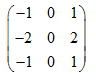deutsch     english    français     Print

## 3.11 IMAGE PROCESSING### INTRODUCTION

 We understand a picture as a flat, rectangular area on which there are colored forms. In printing and computer technology, one describes an image as a grid-like arrangement of colored dots called pixels. The number of pixels per unit of area is called the image resolution and it is often indicated in dots per inch (dpi). In order to save and process an image on the computer, the color must be defined as a number. There are several possibilities for this, which are called either color metrics or color models. One of the most popular models used is the RGB color model, where the intensity of the three color components red, green, and blue are represented by numbers between 0 (dark) and 255 (light) more... This corresponds to the color perception of the human eye, where three different color receptors, L, M and S cones measure the red,]. The ARGB model includes even another number between 0 and 255 that is the measure of transparency (alpha value) [more... A binary number (32 bit): bit 0..7: blue, bit 8..15: green, bit 16..23: red, bit 24..31: alpha]. In short: A computer image consists of a rectangular array of pixels that are encoded as colors. This is often called a bitmap. PROGRAMMING CONCEPTS: Image digitalization, resolution, color model, bitmap, image format

### COLOR MIXING IN THE RGB MODEL

 TigerJython provides you with objects of the type GBitmap, to simplify your work with bitmaps. Using bm = GBitmap(width, height) you produce a bitmap with the desired number of horizontal and vertical pixels. Afterwards, you can set the color of the individual pixels using the method setPixelColor(x, y, color) and read them using getPixelColor(x, y). With the method image() you can finally represent the bitmap in GPanel. Your program will draw the famous 3 circles of additive color mixing as you run through the bitmap with a nested for loop.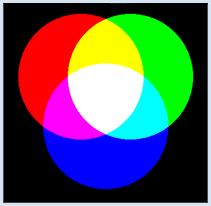```from gpanel import *

xRed = 200
yRed = 200
xGreen = 300
yGreen = 200
xBlue = 250
yBlue = 300

makeGPanel(Size(501, 501))
window(0, 501, 501, 0)    # y axis downwards
bm = GBitmap(500, 500)
for x in range(500):
for y in range(500):
red = green = blue = 0
if (x - xRed) * (x - xRed) + (y - yRed) * (y - yRed) < 16000:
red = 255
if (x - xGreen) * (x - xGreen) + (y - yGreen) * (y - yGreen) < 16000:
green = 255
if (x - xBlue) * (x - xBlue) + (y - yBlue) * (y - yBlue) < 16000:
blue = 255
bm.setPixelColor(x, y, makeColor(red, green, blue))

image(bm, 0, 500)
```
Highlight program code (Ctrl+C copy, Ctrl+V paste)

### MEMO

 Colors are defined by their red, green, and blue components. makeColor(red, green, blue) puts these color components together to a color (a color object). For images we typically use an integer coordinate system with the origin in the upper left corner, with the positive y-axis pointing down [more... You can get rounding errors because the GPanel used float coordinates].

### MAKING A GRAYSCALE IMAGE

 At some point, you may have been asked how your image processing software (such as Photoshop, etc.) actually works. Here, you will get to know some of the simple procedures. Your program can turn a color image into a grayscale image by determining the average of the red, green, and blue components, and then use these to define the gray value.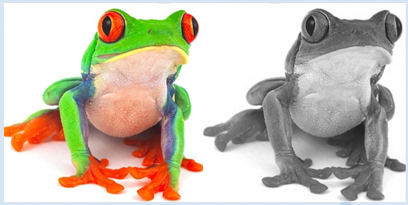```from gpanel import * size = 300 makeGPanel(Size(2 * size, size)) window(0, 2 * size, size, 0) # y axis downwards img = getImage("sprites/colorfrog.png") w = img.getWidth() h = img.getHeight() image(img, 0, size) for x in range(w): for y in range(h): color = img.getPixelColor(x, y) red = color.getRed() green = color.getGreen() blue = color.getBlue() intensity = (red + green + blue) // 3 gray = makeColor(intensity, intensity, intensity) img.setPixelColor(x, y, gray) image(img, size, size) ``` Highlight program code (Ctrl+C copy, Ctrl+V paste)

### MEMO

 You can determine the color values as integers from a color object using the methods getRed(), getGreen(), getBlue(). The background has to be white, not transparent. If you want to allow transparency, you can determine the transparency value with alpha = getAlpha() and then use it in a extra parameter of makeColor(red, green, blue, alpha).

### REUSABILITY

In many image processing programs the user has to be able to select a portion of the image. For this, you can create a temporary rectangle by clicking and dragging the mouse (a "rubber band rectangle"). Once you release the mouse button, the rectangular area will be definitely chosen. It is smart to solve this subproblem first, since its code can be used again later in many other image processing applications. Reusability is a sign of quality in all software development.

As you saw earlier, you can regard the drawing of the rubber band lines as an animation. In this case, however, the entire image needs to be rebuilt with each movement. A neat trick to avoid this is the XOR drawing mode. In this mode, a new figure is combined with the one underneath it so that the figure will be deleted again upon further repainting, without changing the underlying image. The disadvantage to this process is that the colors change while the figure is drawn. However, this does not usually matter in connection with rubber band rectangles.

 The program framework should only call the function doIt() after the rectangle selection, and write the coordinates of the upper left corner ulx (upper left x), uly (upper left y) and the lower right corner lrx (lower right x), lry (lower right y). You will later insert your code for image processing in doIt(). You should be able to understand the code with your previous experience in the chapter about mouse events without any major problems.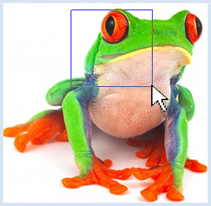```from gpanel import *

size = 300

def onMousePressed(e):
global x1, y1
global x2, y2
setColor("blue")
setXORMode(Color.white) # set XOR paint mode
x1 = x2 = e.getX()
y1 = y2 = e.getY()

def onMouseDragged(e):
global x2, y2
rectangle(x1, y1, x2, y2) # erase old
x2 = e.getX()
y2 = e.getY()
rectangle(x1, y1, x2, y2) # draw new

def onMouseReleased(e):
rectangle(x1, y1, x2, y2) # erase old
setPaintMode() # establish normal paint mode
ulx = min(x1, x2)
lrx = max(x1, x2)
uly = min(y1, y2)
lry = max(y1, y2)
doIt(ulx, uly, lrx, lry)

def doIt(ulx, uly, lrx, lry):
print "ulx = ", ulx, "uly = ", uly
print "lrx = ", lrx, "lry = ", lry

x1 = y1 = 0
x2 = y2 = 0

makeGPanel(Size(size, size),
mousePressed = onMousePressed,
mouseDragged = onMouseDragged,
mouseReleased = onMouseReleased)
window(0, size, size, 0)    # y axis downwards

img = getImage("sprites/colorfrog.png")
image(img, 0, size)
```
Highlight program code (Ctrl+C copy, Ctrl+V paste)

### MEMO

 You can get the bitmap for a picture that you have already stored on your computer by using getImage(), where you must specify the fully qualified name, or just a part of the path relative to the directory in which your program is located. For images located in the distribution, you use the directory name sprites. In the press event, you put the system into XOR mode, so that in your drag event handling you can delete the old rectangle by drawing it a second time, and then draw the new one. You must store the vertices in the global variables x1, y1, x2, y2. If you draw the rubber band rectangle again with the release event before you switch to paint mode, the rectangle will disappear. If you switched to paint mode first, the rectangle would stay. The program will work no matter how you decide to draw the rectangle. It always returns the correct values for ulx,uly and lrx, lry (always ulx < lrx, uly < lry). Be aware that you do not need to convert the mouse coordinates to window coordinates, since both are equal if you are using the same values for the window size with size() and the coordinate system with window(). You still get drag events if you move the mouse out of the window. You have to be careful of what you do with such coordinates, otherwise the program could crash unexpectedly.

### RED-EYE EFFECT

 Image processing plays a central role in the post-processing of digital photos. There are numerous post-processing programs on the Internet, but you do not need to rely on them because you can now write your own program that will be better suited to your needs, with Python and a healthy degree of imagination and perseverance. Your task below is to write a program that can fix the red-eye effect. This occurs when the back of the eye (fundus) reflects the flash. Here you will use a picture of a frog, since it also has other red spots.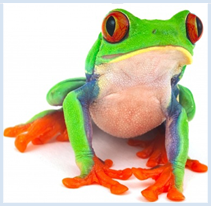```from gpanel import *

size = 300

def onMousePressed(e):
global x1, y1
global x2, y2
setColor("blue")
setXORMode("white")
x1 = x2 = e.getX()
y1 = y2 = e.getY()

def onMouseDragged(e):
global x2, y2
rectangle(x1, y1, x2, y2) # erase old
x2 = e.getX()
y2 = e.getY()
rectangle(x1, y1, x2, y2) # draw new

def onMouseReleased(e):
rectangle(x1, y1, x2, y2) # erase old
setPaintMode()
ulx = min(x1, x2)
lrx = max(x1, x2)
uly = min(y1, y2)
lry = max(y1, y2)

doIt(ulx, uly, lrx, lry)

def doIt(ulx, uly, lrx, lry):
for x in range(ulx, lrx):
for y in range(uly, lry):
col = img.getPixelColor(x, y)
red = col.getRed()
green = col.getGreen()
blue = col. getBlue()
col1 = makeColor(3 * red // 4, green, blue)
img.setPixelColor(x, y, col1)
image(img, 0, size)

x1 = y1 = 0
x2 = y2 = 0

makeGPanel(Size(size, size),
mousePressed = onMousePressed,
mouseDragged = onMouseDragged,
mouseReleased = onMouseReleased)
window(0, size, size, 0)    # y axis downwards

img = getImage("sprites/colorfrog.png")
image(img, 0, size)
```
Highlight program code (Ctrl+C copy, Ctrl+V paste)

### MEMO

 The code for processing the image is latched in the function doIt()You can take everything else unchanged from the previous program. You can adjust the degree of attenuation of the color red. Here, the red intensity is set down to 75%. Be aware of the double slash, which performs an integer division (the remainder is ignored). The result is again an integer, just as it should be. The program still shows some errors which you can easily fix. Firstly, it also discolors non-red areas, and secondly, it crashes when you pull the rubber band rectangle out of the window. Of course it would be really nice if the program could find the red eyes itself. However, to do this it would have to analyze the image and recognize its contents automatically, which is an especially challenging problem in computer science [more... Image recognition is a branch of the high current pattern recognition].

### CUTTING AND STORING PICTURES

 Cutting images is also one of the basic functions of image processing programs. Your program can not only copy a selected part of the image to another window using the rubber band rectangle, but it can also store this image as a JPEG file for future use.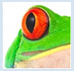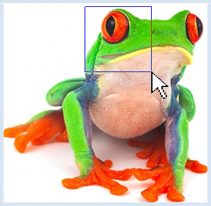```from gpanel import *

size = 300

def onMousePressed(x, y):
global x1, y1
global x2, y2
setColor("blue")
setXORMode("white")
x1 = x2 = int(x)
y1 = y2 = int(y)

def onMouseDragged(x, y):
global x2, y2
rectangle(x1, y1, x2, y2) # erase old
x2 = int(x)
y2 = int(y)
rectangle(x1, y1, x2, y2) # draw new

def onMouseReleased(x, y):
rectangle(x1, y1, x2, y2) # erase old
setPaintMode()
ulx = min(x1, x2)
lrx = max(x1, x2)
uly = min(y1, y2)
lry = max(y1, y2)
doIt(ulx, uly, lrx, lry)

def doIt(ulx, uly, lrx, lry):
width = lrx - ulx
height = lry - uly
if ulx < 0 or uly < 0 or lrx > size or lry > size:
return
if width < 20 or height < 20:
return

cropped = GBitmap.crop(img, ulx, uly, lrx, lry)
p = GPanel(Size(width, height))  # another GPanel
p.window(0, width, 0, height)
p.image(cropped, 0, 0)
rc = save(cropped, "mypict.jpg", "jpg")
if rc:
p.title("Saving OK")
else:
p.title("Saving Failed")

x1 = y1 = 0
x2 = y2 = 0

makeGPanel(Size(size, size),
mousePressed = onMousePressed,
mouseDragged = onMouseDragged,
mouseReleased = onMouseReleased)
window(0, size, size, 0)    # y axis downwards

img = getImage("sprites/colorfrog.png")
image(img, 0, size)
```
Highlight program code (Ctrl+C copy, Ctrl+V paste)

### MEMO

 You can view more than one GPanel window if necessary, by creating GPanel objects. To draw, use the graphics commands which you call using the point operator. If the selected section is too small (especially if you click with the mouse without dragging), doIt() ends with an empty return, and likewise if the vertices are not in the image area. To save, use the method save(), where the last parameter determines the image format. The allowed values are: "bmp", "gif", "jpg", "png".

### EXERCISES

1.
 Write a program that swaps the red and green components of the image colorfrog.png.

2.
 Write a program where you can rotate the image by dragging the mouse. Use the function atan2(y, x) which provides you with the angle α to the point P(x, y). You still have to convert this to degrees using math.degrees() before you can rotate the picture with GBitmap.scale(). You can take colorfrog.png as a test image again.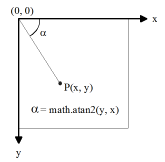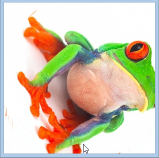3.
 Write a photo retouching program that can store the color of a pixel with a click of the mouse (color picker). The following dragging should draw colored circles filled this way into the image. Here you have to use the press, drag, and click events. You can again use colorfrog.png as a test image. Write the 3 color components of the "picked" color in the title bar of the window.

### FILTERING IMAGES WITH CONVOLUTION

You surely know that in conventional image processing programs you are able to modify an image with various filters, such as smoothing filters, sharpening filters, blurring filters, etc. Here, the important principle of convolution is used, which you can learn about [more... Convolution is a principle that often be used in the mathematics, in the natural sciences and in the engineering]. In this process, you change the color values of each pixel by calculating a new value from it and its eight neighboring pixels, according to a filtering rule.

In detail, this works as follows: For the sake of simplicity, consider a greyscale image where each pixel in the RBG coloring model possesses a gray value v between 0 and 255. The filtering rule is defined by nine numbers that are arranged in a square:

m00   m01   m02
m10   m11   m12
m20   m21   m22

 This representation is called a convolution matrix (also called mask). In Python we implement it line by line in a list mask = [[0, -1, 0], [-1, 5, 1], [0, -1, 0]] With this data structure you can easily access the individual values with double indices, for example m12 = mask = 1. These nine numbers are weighting factors for a pixel and its eight neighbors. Now you can calculate the new gray value vnew of a pixel at the point x, y from the existing nine values v(x, y) as follows: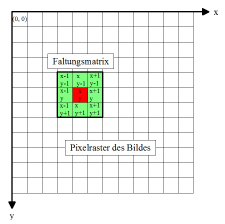vnew(x, y) = m00 * v(x - 1, y -1) + m01 * v(x, y - 1) + m02 * v(x + 1, y - 1) + m10 * v(x - 1, y) + m11 * v(x, y) + m12 * v(x + 1, y) + m20 * v(x - 1, y + 1) + m21 * v(x , y + 1) + m22 * v(x + 1, y + 1)

To illustrate, one could say that for the recalculation one places the convolution matrix above the pixel, multiplies its values with the underlying gray values, and finally sums them all up.

The program performs these convolution operations for all of the pixels (except the boundary points) and then saves the resulting gray values in a new bitmap, which it then displays. To do this you move the convolution matrix row by row, from left to right and from top to bottom, over the image with a for structure. Here you use the convolution matrix values of a sharpening filter and the grayscale image frogbw.png of the frog.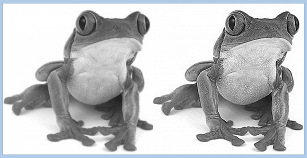```from gpanel import *

size = 300

makeGPanel(Size(2 * size, size))
window(0, size, size, 0)    # y axis downwards

bmIn = getImage("sprites/frogbw.png")
image(bmIn, 0, size)
w = bmIn.getWidth()
h = bmIn.getHeight()
bmOut = GBitmap(w, h)

#mask = [[1/9, 1/9, 1/9], [1/9, 1/9, 1/9], [1/9, 1/9, 1/9]]  # smoothing
mask = [[ 0, -1,  0], [-1,  5, -1], [0,  -1,  0]] #sharpening
#mask = [[-1, -2, -1], [ 0,  0,  0], [ 1,  2,  1]] #horizontal edge extraction
#mask = [[-1,  0,  1], [-2,  0,  2], [-1,  0,  1]] #vertical edge extraction

for x in range(0, w):
for y in range(0, h):
if x > 0 and x < w - 1 and y > 0 and y < h - 1:
vnew = 0
for k in range(3):
for i in range(3):
c = bmIn.getPixelColor(x - 1 + i, y - 1 + k)
v = c.getRed()
vnew +=  v * mask[k][i]
# Make int in 0..255
vnew = int(vnew)
vnew = max(vnew, 0)
vnew = min(vnew, 255)
gray = Color(vnew, vnew, vnew)
else:
c = bmIn.getPixelColor(x, y)
v = c.getRed()
gray = Color(v, v, v)

bmOut.setPixelColor(x, y, gray)

image(bmOut, size / 2, size)
```
Highlight program code (Ctrl+C copy, Ctrl+V paste)

### MEMO

 In a convolution, each pixel is replaced by a weighted average of itself and its neighboring points. The filter type is determined by the convolution matrix.

You can experiment with the following well-known convolution matrices, or you can invent your own.

Filter type Convolution matrix
Sharpening filter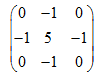Smoothing filter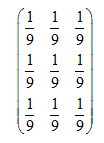Edge extraction (horizontal)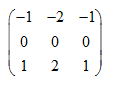Edge extraction (vertical)